Home > CCA2 > Chapter 8 > Lesson 8.1.1 > Problem8-8

8-8.
1. For each equation below, make tables that include x-values from −2 to 2 and draw each graph. Homework Help ✎

1. y = (x − 1)2(x + 1)

2. y = (x − 1)2(x + 1)2

3. y = x3 − 4x

4. What are the parent functions for these equations?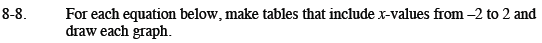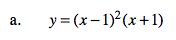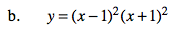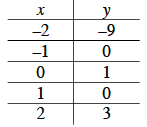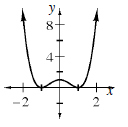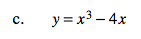Use the same method as in parts (a) and (b).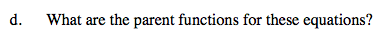What is the highest-valued exponent?

y = (x − 1)2 (x + 1)

y = (x2 − 2x + 1) (x + 1)

y = x3x2x + 1

The parent function is y = x3.